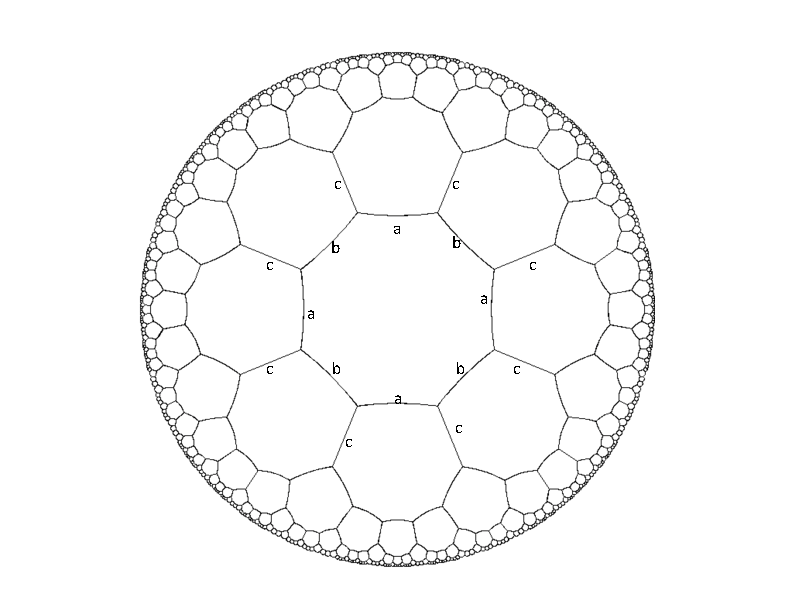시간 제한메모리 제한제출정답맞힌 사람정답 비율
1 초 128 MB106660.000%

## 문제

Below is a picture of an infinite hyperbolic tessellation of octagons. If we think of this as a graph of vertices (of degree three), then there exists an isomorphism of the graph which maps any vertex x onto any other vertex y. Every edge is given a label from the set {a,b,c} in such a way that every vertex has all three types of edges incident on it, and the labels alternate around each octagon. Part of this labeling is illustrated in the diagram.So a path in this graph (starting from any vertex) can be specified by a sequence of edge labels. Your job is to write a program which, given a squence of labels such as "abcbcbcabcaccabb", returns "closed" if the path ends on the same vertex where it starts, and returns "open" otherwise.

## 입력

The input will begin with a number Z ≤ 200 on a line by itself. This is followed by Z lines, each of which is a squence of length at least 1 and at most 40 of 'a's 'b's and 'c's.

## 출력

For each input instance, the output will be the words "closed" or "open", each on a single line.

## 예제 입력 1

2
abababab
abcbcbcbcba


## 예제 출력 1

closed
open